Question

Fluid Mechanics

A vertical cylindrical tank with a 0.4 m diameter circular base holds water, the level of which is a height of 1.3 m from the bottom of the tank. If the tank has a 2.5 cm diameter orifice at its base, calculate the distance the level of water will be from the base of the tank after300 seconds.

\text { Given: } c_{d}=0.5 \text { for the orifice }

\text { Given: } T=\int_{H_{2}}^{H_{1}} \frac{A d h}{C_{d} a \sqrt{2 g h}}

where the symbols have their usual meaning.

A dam is shown in Figure Q2(b). Given the dimensions shown in the diagram, calculate:

(i)The resultant force from the water on the dam per unit width.

(ii)The direction of the resultant force.

A horizontal Venturi meter, pipe diameter of 500 mm and throat diameter of 280 mm, has a discharge coefficient of 0.97. A differential U-tube manometer, using mercury as the manometric fluid, is connected between pressure tappings at the entrance and atthe throat. The Venturi meter is used to measure the flow of water,which fills the leads to the U-tube and is in contact with the mercury.

(i) Calculate the volume flow rate when the difference in the mercury levels is 50 mm. Take densities of water and mercury as 1000 kg/m³ and 13,600 kg/m³ respectively.

\text { Given: } v_{1}=\sqrt{\frac{2 g x\left(\frac{\rho_{m}-\rho_{p}}{\rho_{p}}\right)}{\left(\frac{a_{1}}{a_{2}}\right)^{2}-1}}

\text { Given: } Q=v_{1} \cdot a_{1} \cdot c_{d}

where the symbols have their usual meaning.

In less than 100 words describe the Velocity Pressure and Static Pressure changes as the fluid flows through the meter according to Bernoulli's equation.

A rectangular pontoon floating in salt water of density 1025 kg/m³ is carrying a load. The combined mass of the load and the pontoon is75000 kg. The dimensions of the pontoon are 15 m long, 6 m wide and 2 m high.

Calculate the depth of immersion and freeboard of the pontoon.Verified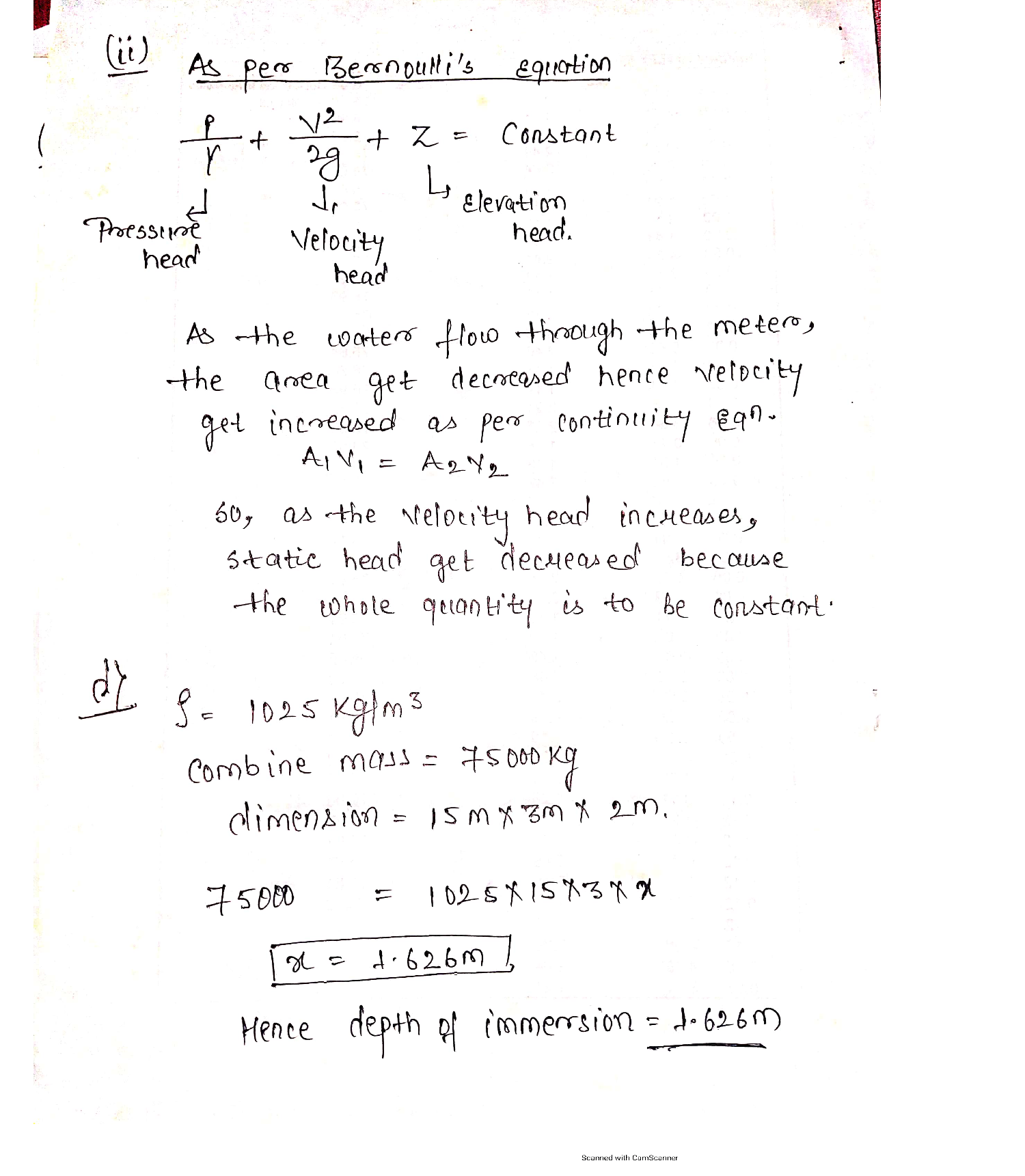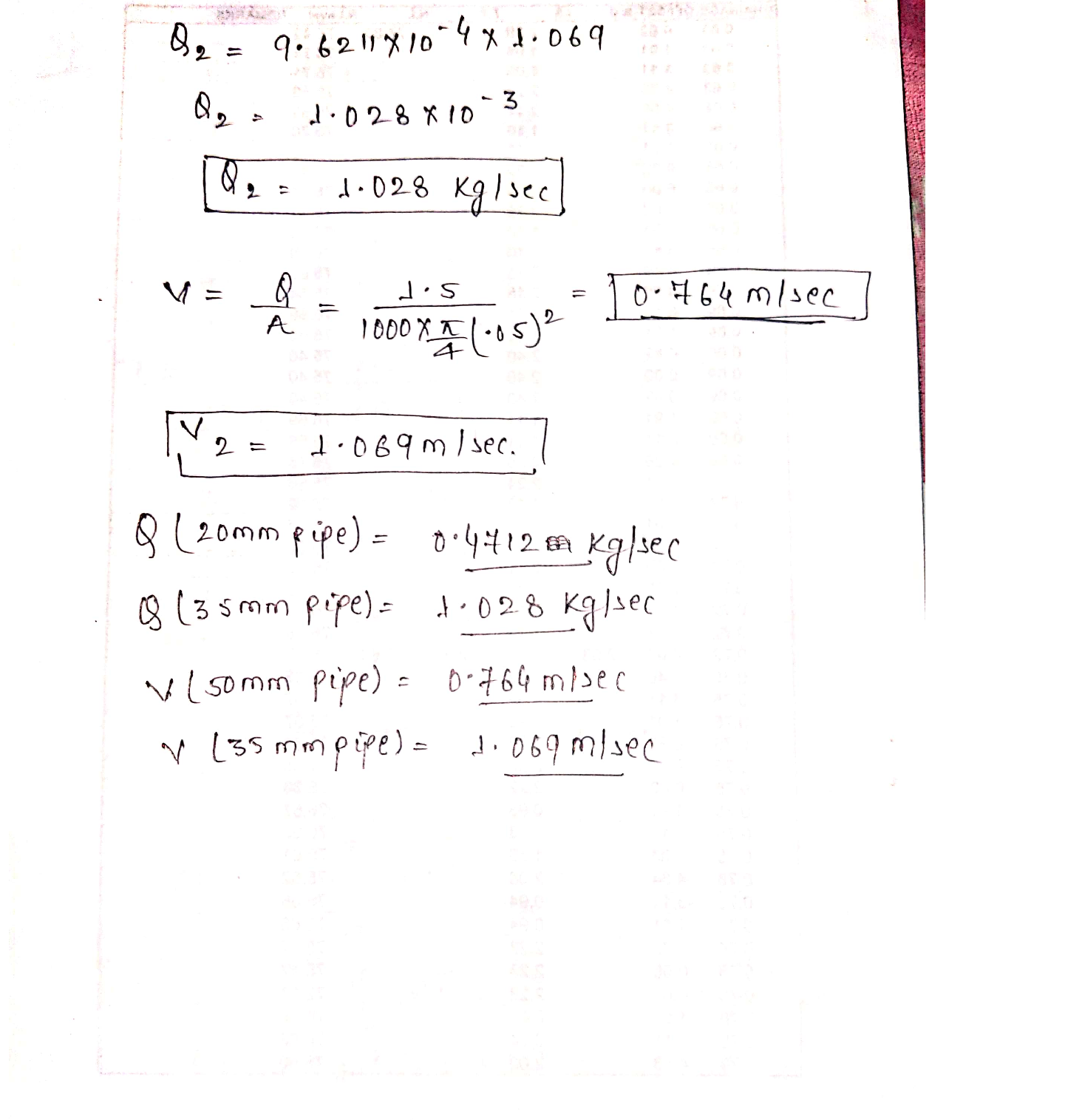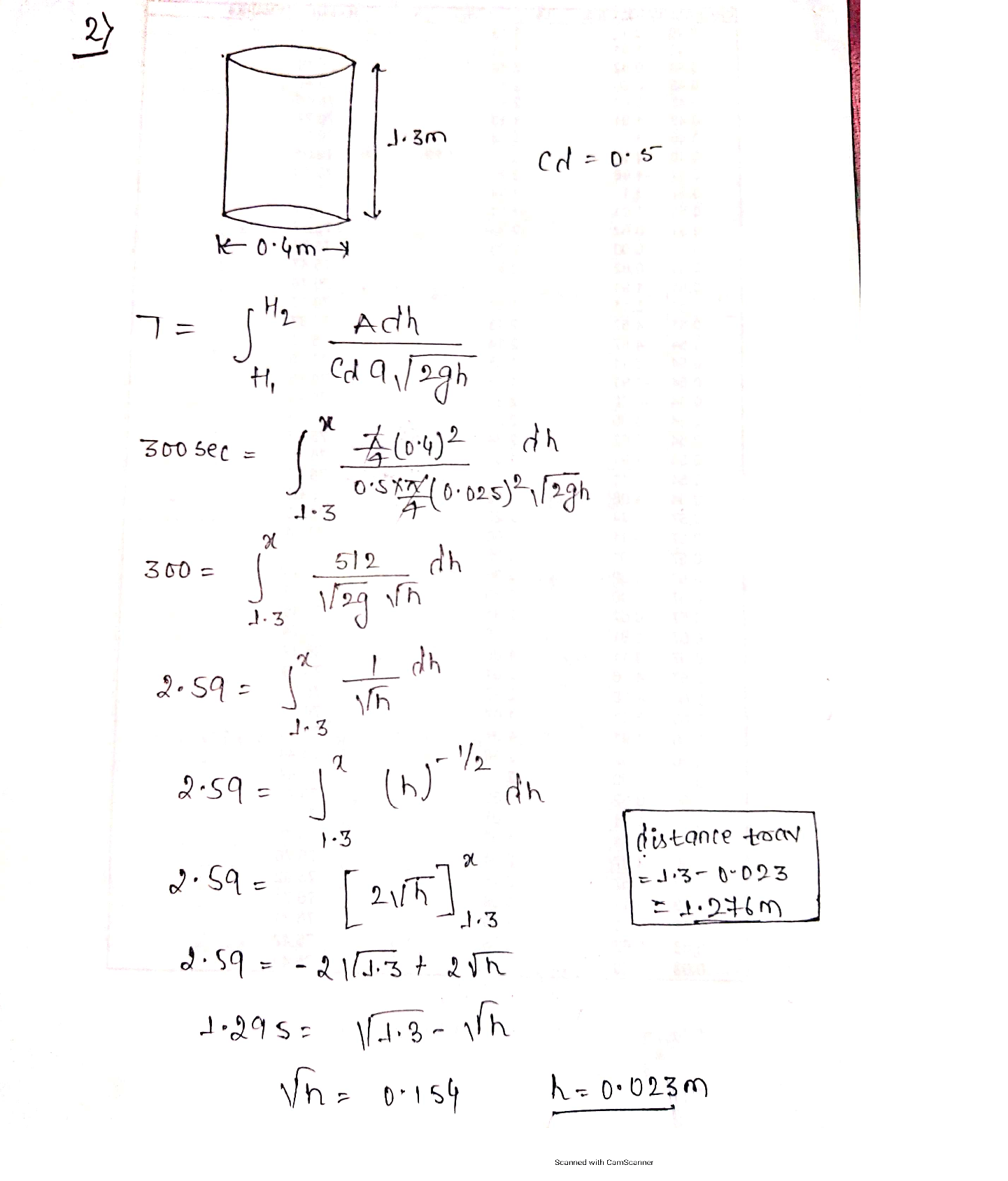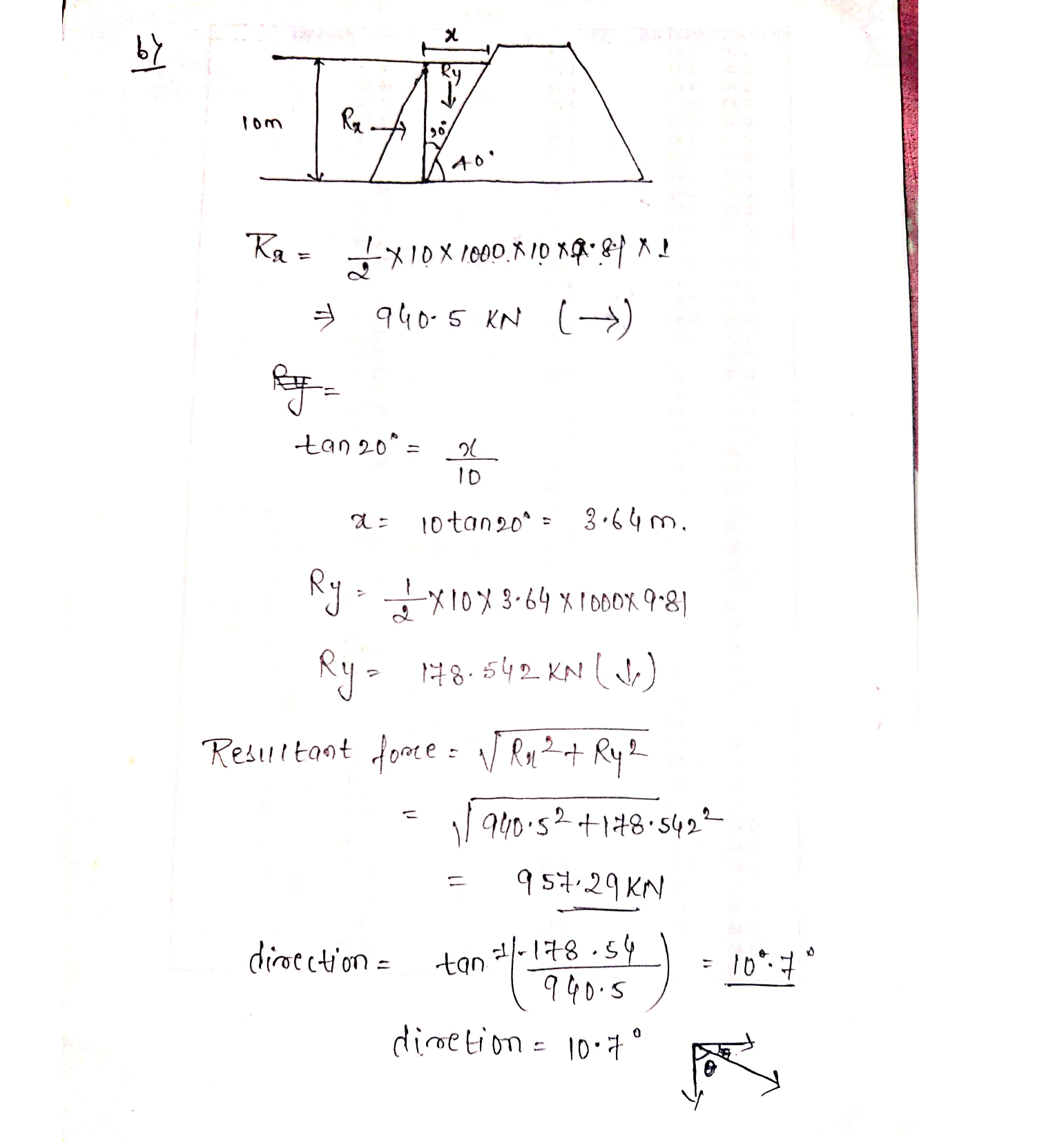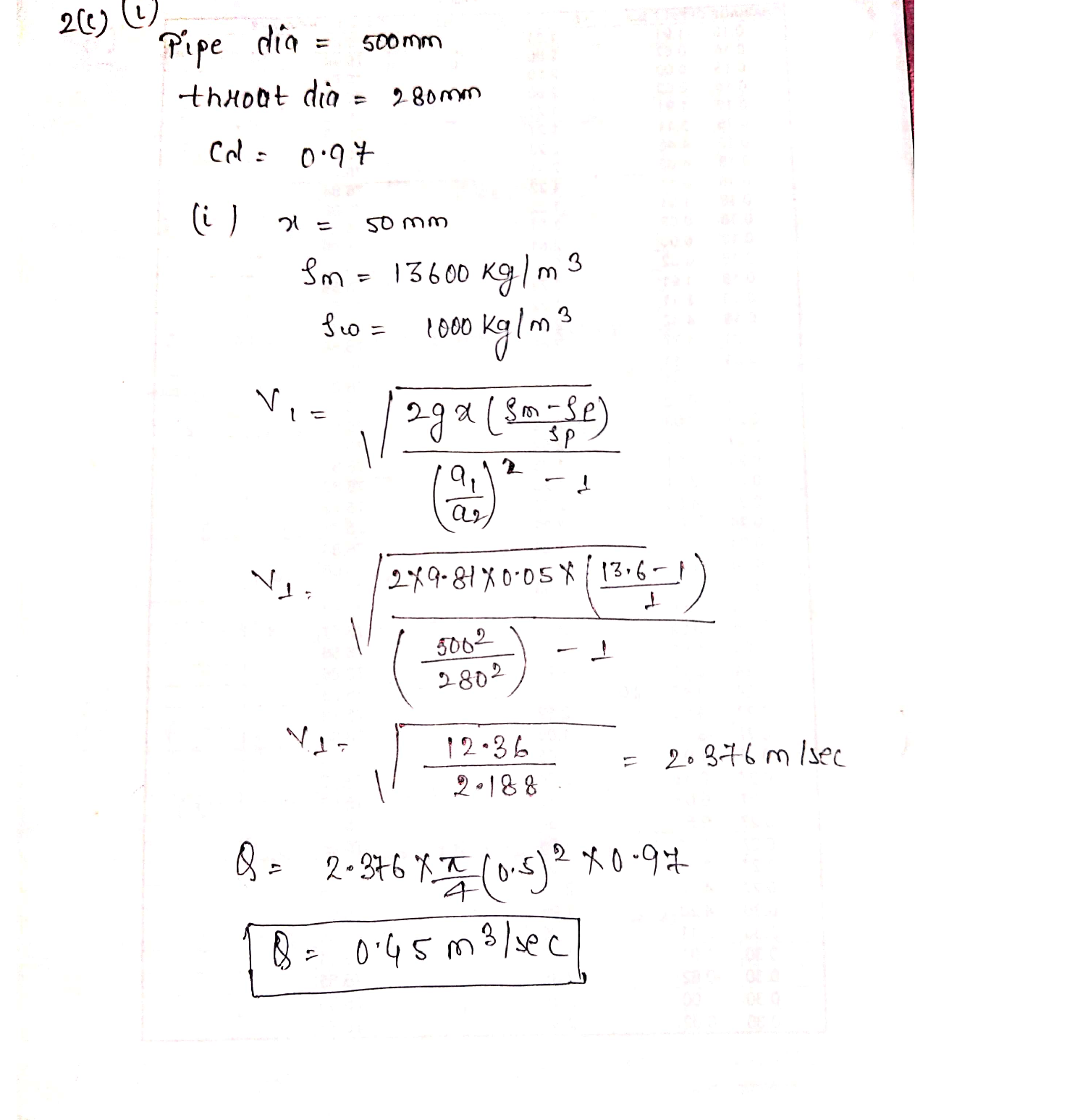### Question 45484Fluid Mechanics

Water in the Belle Fourche Reservoir in Western South Dakotais transported to the water storage reservoir for the town of-Buffalo through a series of three pipes, all with friction factors of 0.02. The first pipe is 500 meters long with a diameter of 0.4meters; the second pipe is 600 meters long with a diameter of0.3 meters; and the third pipe is 500 meters long with a diameter of 0.2 meters. The water surface elevation of the Belle FourcheReservoir is 30 meters higher than the town's storage reservoir.Since the current piping is almost 60 years old, the town of Buffalo has decided to replace the piping system with a single pipe of constant diameter. You have been chosen to calculate the equivalent diameter for the single pipe design and determine the flow rate that will occur. Go to work.

### Question 44899Fluid Mechanics

Transmission lines

### Question 44896Fluid Mechanics

A boat has a volume of 40.0 m³ and a mass of 2.00 tons. What load will push 75.0% of its volume into water? Each Metric ton is 1000 kg.

### Question 44895Fluid Mechanics

A tank has a height of 800 mm and a length of 500 mm. The tank contains a liquid of RD 0.9.Determine:
a. The magnitude of the force on the side of the tank per meter width and its position relative to the base of the tank
b. The force on the base per meter width of tank.

### Question 44894Fluid Mechanics

A closed tank contains three different fluids with a vapor space above the oil, as shown in the figure below. Pressure gauge 1 reads the gauge pressure of the vapor space (P1) and pressure gauge 2 reads the pressure at the bottom of the tank (P2). When P1 = 25 kPa, determine:
a. Height of glycerine (h) in the tank if P2 = 95.66 kPa
. P2 reading if glycerine is replaced with water

### Question 44805Fluid Mechanics

Water flows into cylindrical tank through inlet A and D at rate of 20 ft/s and 5 ft/s respectively. If waterleaves through outlet B and C at rate of 3 ft/s and 10 ft/s respectively.
(A) Derive the continuity equation
\text { Know that } D_{A}=2.5 \text { in, } D_{D}=2 \text { in } D_{B}=3 \text { in and } D_{C}=3.5 \text { in. }
(B) Calculate the change of high h?

### Question 44804Fluid Mechanics

An object which has cubic shape is been floating on the water surface see the diagram below. If the object density is less than water, density find the ratio X/L?

### Question 44803Fluid Mechanics

A force of 15.83 kN is exerted on a piston B (diameter = 250 mm) which fits into a cylinder see the diagram below. How much force is need on piston C to start displacing piston B and Mercury on piston B?

### Question 44802Fluid Mechanics

Given the diagram below if the pressure gauge reads 6.1 psig find Y and X in ft. Given that
\mathrm{SG}_{\mathrm{Aloohal}}=0.9, \mathrm{SG}_{\mathrm{Hg}}=13.6, \text { Alcohol vapor } P=1.7 \mathrm{psig}, Z=4 \mathrm{ft} \text { and } \mathrm{Y}_{\mathrm{H} 2 \mathrm{o}}=62.4 \mathrm{\textrm {lb }} / \mathrm{ft}^{3}

### Question 44801Fluid Mechanics

Given the diagram below, calculate the pressure at A, B, C.
\text { Given that } \mathrm{Y} \text { н2о }=9.79 \mathrm{kN} / \mathrm{m}

### Submit query